# Descent, method of

(diff) ← Older revision | Latest revision (diff) | Newer revision → (diff)

A method for solving the minimization problem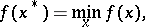whereis some function of the variables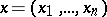. The iterative sequence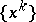of the method of descent is computed by the formula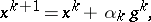where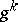is a vector indicating some direction of decrease ofat, and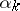is an iterative parameter, the value of which indicates the step-length in the direction. Ifis a differentiable function and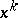is not an extremal point of it, then the vectormust satisfy the inequality(*)

where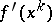is the gradient ofat.

Ifis a sufficiently smooth function (e.g. twice continuously differentiable) and if the sequence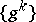satisfies inequality (*), then there exists a sequence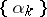such that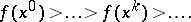Under certain restrictions (see ) on the functionand on the method of choosing the parametersand the vectors, the sequenceconverges to a solutionof the initial problem.

The gradient method, in which the vectorsare in some way expressed in terms of the vectors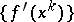, is a method of descent. One of the most common cases is whenwhere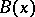is a symmetric matrix satisfyingfor any two vectorsand, with certain constants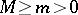. Under additional restrictions (see ) onand by a special selection of, the gradient method ensures the convergence ofto a solutionof the initial problem with the rate of an arithmetical progression with ratio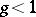. A special case of the gradient method is the method of steepest descent (cf. Steepest descent, method of), in which the matrixis the unit matrix.

How to Cite This Entry:
Descent, method of. Encyclopedia of Mathematics. URL: http://encyclopediaofmath.org/index.php?title=Descent,_method_of&oldid=11500
This article was adapted from an original article by Yu.A. Kuznetsov (originator), which appeared in Encyclopedia of Mathematics - ISBN 1402006098. See original article